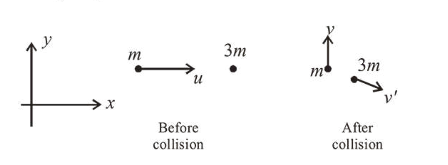# A particle of mass m with an initial velocityQuestion:

A particle of mass $m$ with an initial velocity $u \hat{i}$ collides perfectly elastically with a mass $3 \mathrm{~m}$ at rest. It moves with a velocity $v \hat{j}$ after collision, then, $v$ is given by:

1. (1) $v=\sqrt{\frac{2}{3}} u$

2. (2) $v=\frac{u}{\sqrt{3}}$

3. (3) $v=\frac{u}{\sqrt{2}}$

4. (4) $v=\frac{1}{\sqrt{6}} u$

Correct Option:

Solution:

(3) From conservation of inear momentum

$m u \hat{i}+0=m v \hat{j}+3 m \overrightarrow{v^{\prime}}$

$\overrightarrow{v^{\prime}}=\frac{u}{3} \hat{i}-\frac{v}{3} \hat{j}$From kinetic energy conservation,

$\frac{1}{2} m u^{2}=\frac{1}{2} m v^{2}+\frac{1}{2}(3 m)\left(\left(\frac{u}{3}\right)^{2}+\left(\frac{v}{3}\right)^{2}\right)$

or, $m u^{2}=m v^{2}+\frac{m u^{2}}{3}+\frac{m v^{2}}{3}$

$\therefore v=\frac{u}{\sqrt{2}}$